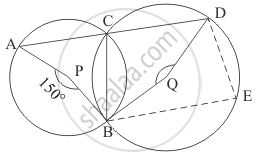Advertisement Remove all ads

# In the Given Figure, P and Q Are Centres of Two Circles Intersecting at B and C. Acd is a Straight Line. Then, ∠Bqd = - Mathematics

Answer in Brief

In the given figure, P and Q are centres of two circles intersecting at B and CACD is a straight line. Then, ∠BQD =Advertisement Remove all ads

#### Solution

Consider the circle with the centre ‘P’.

The angle subtended by an arc at the centre of the circle is double the angle subtended by the arc in the remaining part of the circle.So, here we have

angleACB = (angleAPB )/2

=(150°)/2

angleACB  = 75°

Since ‘ACD’ is a straight line, we have

angleACB + angleBCD = 180°

angleBCD = 180° - angleACB

= 180° - 75°

angleBCD  = 105°

Now let us consider the circle with centre ‘Q’. Here let ‘E’ be any point on the circumference along the major arc ‘BD’. Now ‘CBED’ forms a cyclic quadrilateral.

In a cyclic quadrilateral it is known that the opposite angles are supplementary, meaning that the opposite angles add up to 180°.

So here,

angleBCD + angleBED  = 180°

angleBED = 180° - angleBCD

= 180° - 105°

angleBED = 75°

The angle subtended by an arc at the centre of the circle is double the angle subtended by the arc in the remaining part of the circle.

So, now we have

angleBQD = 2 angleBED

=2(75°)

angleBQD = 150°

Hence, the measure of  angleBQD is  150° .

Is there an error in this question or solution?
Advertisement Remove all ads

#### APPEARS IN

RD Sharma Mathematics for Class 9
Chapter 15 Circles
Q 7 | Page 108
Advertisement Remove all ads

#### Video TutorialsVIEW ALL 

Advertisement Remove all ads
Share
Notifications

View all notifications

Forgot password?
Course